### Home > CC3 > Chapter 2 > Lesson 2.1.4 > Problem2-44

2-44.

Copy and complete each of the Diamond Problems below. The pattern used in the Diamond Problems is shown below.  Homework Help ✎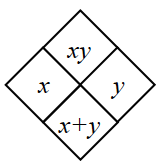1.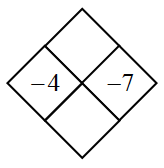Add $x$ and $y$ to fill the bottom and multiply them to fill the top.

Bottom: $−11$
Top: $28$

1.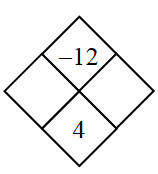What are the factors of $−12$?

Which of those add to $4$?

The larger factor must be positive in order for the sum to be positive.

1.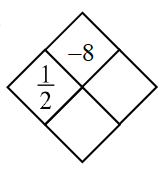Divide $8$ by $-\frac{1}{2}$ to get $y$

1.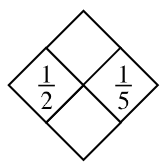Follow the steps in part (a).

Top: $\frac{1}{10}$

Bottom: $\frac{7}{10}$